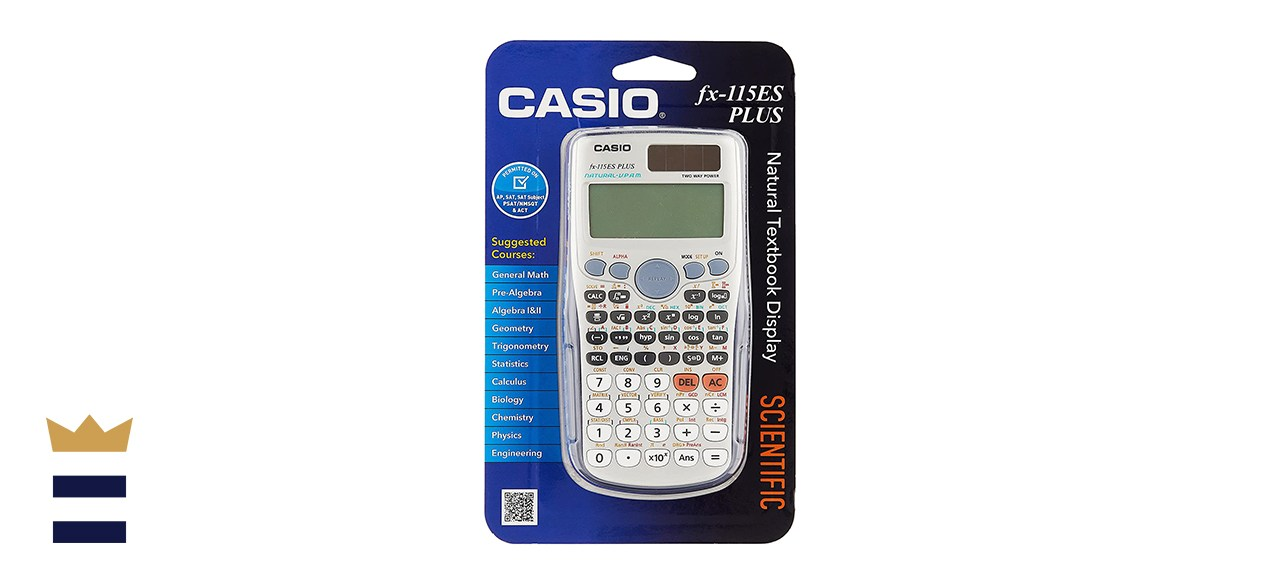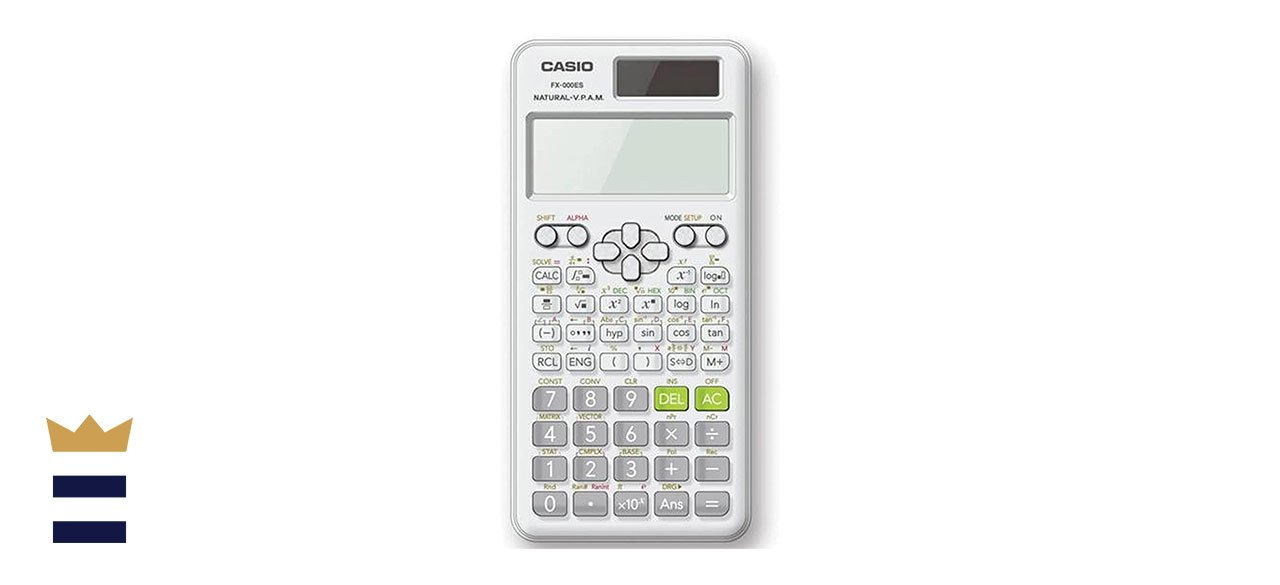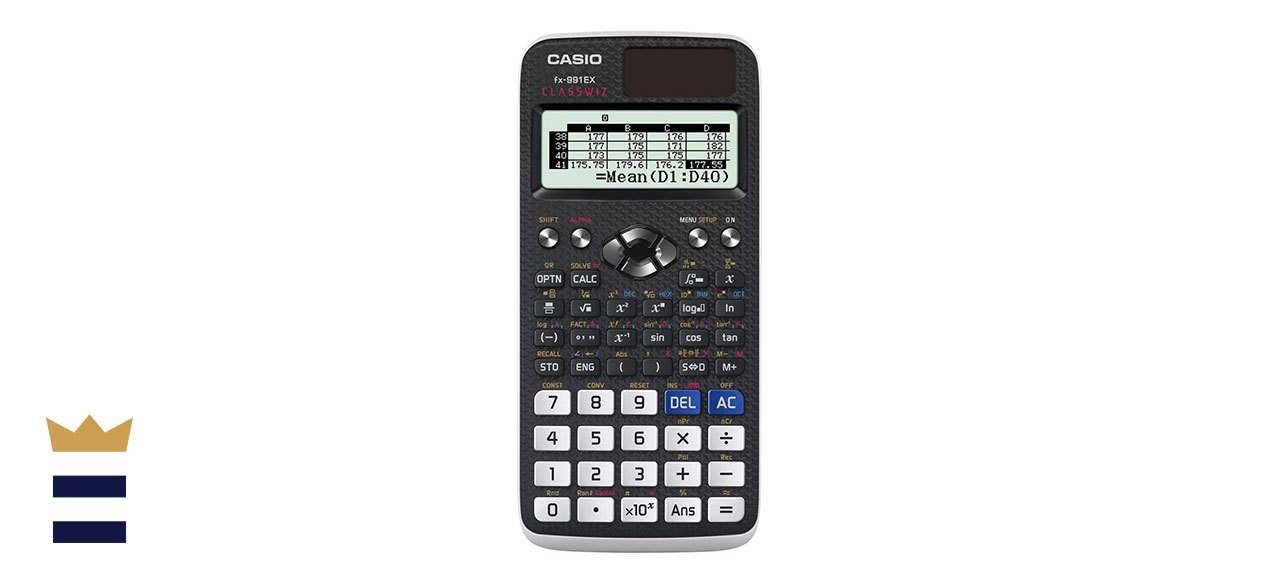# Best Casio scientific calculator

EducationBestReviews is reader-supported and may earn an affiliate commission. Details.

## Which Casio scientific calculators are best?

You will probably need a Casio scientific calculator at some point in your educational journey to help you figure out formulas, equations, variables, and functions more quickly. You can solve advanced problems in a jiffy in physics, mathematics and engineering with a number of different Casio scientific calculators on the market.

The Casio FX-115ESPLUS Engineering/Scientific Calculator is a first-rate choice for a Casio scientific calculator with easy, intuitive access to commands.

## What to know before you buy a Casio scientific calculator

### Learn about the different kinds of calculators

There are a few different kinds of calculators, including graphing calculators, scientific calculators and basic calculators. Basic calculators only do basic math functions, like division, multiplication, subtraction and addition, but some of these calculators might be able to do square roots and percentages as well.

Scientific calculators can perform functions like logarithms, tangents, sines and cosines. The more advanced scientific calculators have multi-function keys and can solve true or false questions and variable equations. Graphic calculators have many of the same abilities and features as scientific calculators, but they allow you to view the solution in graph form.

### Understand the various power options for Casio scientific calculators

There are also a few different power options for Casio scientific calculators, including dual power, solar power and battery power. Battery-powered scientific calculators use either disposable or rechargeable batteries, while solar-powered scientific calculators charge from artificial or natural light. Dual-powered scientific calculators mix both battery and solar power sources, allowing you to use solar power if your battery runs out.

### Find the right cover for your Casio scientific calculator

It’s important to add a lid, case or cover to your Casio scientific calculator to protect both the calculator screen and the keys, especially if you are going to be regularly throwing your calculator into a bag, backpack or pocket.

## What to look for in a quality Casio scientific calculator

### Multi-function keys

Multi-function keys enable you to perform multiple functions with the same key. This allows you to solve a diverse range of different complex math problems with a manageably small number of keys or buttons.

### Multi-line screen

Multi-line screens on Casio scientific calculators allow you to view more of what you’ve entered into the calculator, which can reduce the opportunity for making a mistake. In addition, you can see both the math problem and the math problem’s solution simultaneously, which helps you better understand all of the math concepts involved.

### Replay

The replay function on your Casio scientific calculator enables you to go backward or forward through all of your calculations to double- and triple-check your work. If you do notice a mistake, you can correct it and move forward without starting over from the beginning.

## How much you can expect to spend on a Casio scientific calculator

Casio scientific calculators range in price from about \$8-\$100, depending on the features and quality of the calculator.

## Casio scientific calculator FAQ

### How does a Casio scientific calculator work?

A. Electronic language tends to be binary, which means that the language is based on two different things, including off or on, and these things are represented by zeros and ones. Every key that you use on the scientific calculator is then translated into the electronic language.

Casio scientific calculators use a series of routines and rules to instantly process complex computations. The scientific calculator can then translate the electronic language back into characters and numbers on the screen display.

### For which classes in school do you need Casio scientific calculators?

A. Casio scientific calculators are first useful to have in early algebra, and they become more necessary when you start taking higher levels of algebra. These scientific calculators are also helpful in classes like chemistry, biology and statistics.

Scientific calculators can also perform the math you need for classes like engineering, physics, calculus and trigonometry. But you should probably use a graphing calculator for these classes instead since you will likely need to see a visual depiction of the answer in graph form.

### Are you allowed to use a Casio scientific calculator on the SAT?

A. Yes, you can use a Casio scientific calculator on the SAT. Still, you can’t use any calculators that have a QWERTY keyboard, a stylus or power cords or anything that connects to the internet on the SAT.

## What are the best Casio scientific calculators to buy?

### Top Casio scientific calculatorCasio FX-115ESPLUS Engineering/Scientific Calculator

What you need to know: This solid scientific calculator from Casio provides plenty of excellent functions at a fairly affordable price.

What you’ll love: This Casio scientific calculator is perfect for both professionals and students with 280 functions, including statistics, fractions and other calculations. The calculator is also approved for college entrance tests.

What you should consider: The buttons on this Casio scientific calculator sometimes come loose.

Where to buy: Sold by Amazon

### Top Casio scientific calculator for the moneyCasio FX-115ESPLUS2 2nd Edition Advanced Scientific Calculator

What you need to know: This scientific calculator from Casio comes with over 280 functions at a fairly low price.

What you’ll love: This inexpensive Casio scientific calculator features more than 280 functions, including statistics, complex number calculations, fractions, linear regression, computer science, standard deviation, base arithmetic and more.

What you should consider: This second edition Casio scientific calculator doesn’t have many updates.

Where to buy: Sold by Amazon

### Worth checking outCasio FX-991EX Engineering/Scientific Calculator

What you need to know: This scientific calculator from Casio offers a high-resolution LCD screen to reduce the need for scrolling.

What you’ll love: This Casio scientific calculator is an excellent option for geometry, algebra and calculus, with advanced calculations that enable you to work quickly through multi-step math problems.

What you should consider: The numbers on this Casio scientific calculator are smaller, so they are more difficult to read than on other models.

Where to buy: Sold by Amazon